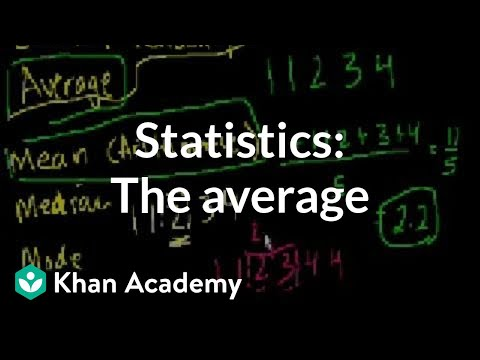# Khan Academy► Play all

# Statistics

• Khan Academy
• 67 videos
• 4,426,889 views
• Last updated on Jul 2, 2014
Introduction to statistics. Will eventually cover all of the major topics in a first-year statistics course (not there yet!)

Loading...

•  Statistics: The average | Descriptive statistics | Probability and Statistics | Khan Academy 12:35 Statistics: Sample vs. Population Mean 6:42 Statistics: Variance of a population | Probability and Statistics | Khan Academy 12:23 Statistics: Sample variance | Descriptive statistics | Probability and Statistics | Khan Academy 11:18 Statistics: Standard deviation | Descriptive statistics | Probability and Statistics | Khan Academy 13:07 Statistics: Alternate variance formulas | Probability and Statistics | Khan Academy 12:17 Introduction to Random Variables 12:04 Probability density functions | Probability and Statistics | Khan Academy 10:02 Binomial Distribution 1 12:16 Binomial Distribution 2 11:05 Binomial Distribution 3 13:27 Binomial Distribution 4 10:46 Expected Value: E(X) 14:53 Expected value of binomial distribution | Probability and Statistics | Khan Academy 16:56 Poisson process 1 | Probability and Statistics | Khan Academy 11:01 Poisson process 2 | Probability and Statistics | Khan Academy 12:42 Law of large numbers | Probability and Statistics | Khan Academy 9:00 Normal distribution excel exercise | Probability and Statistics | Khan Academy 26:04 Introduction to the normal distribution | Probability and Statistics | Khan Academy 26:24 ck12.org normal distribution problems: Qualitative sense of normal distributions | Khan Academy 10:53 ck12.org normal distribution problems: z-score | Probability and Statistics | Khan Academy 7:48 ck12.org normal distribution problems: Empirical rule | Probability and Statistics | Khan Academy 10:25 k12.org exercise: Standard normal distribution and the empirical | Khan Academy 8:16 ck12.org: More empirical rule and z-score practice | Probability and Statistics | Khan Academy 5:57 Central limit theorem | Inferential statistics | Probability and Statistics | Khan Academy 9:49 Sampling distribution of the sample mean | Probability and Statistics | Khan Academy 10:52 Sampling distribution of the sample mean 2 | Probability and Statistics | Khan Academy 13:20 Standard error of the mean | Inferential statistics | Probability and Statistics | Khan Academy 15:15 Sampling distribution example problem | Probability and Statistics | Khan Academy 14:28 Mean and variance of Bernoulli distribution example | Probability and Statistics | Khan Academy 8:20 Bernoulli distribution mean and variance formulas | Probability and Statistics | Khan Academy 6:59 Margin of error 1 | Inferential statistics | Probability and Statistics | Khan Academy 15:02 Margin of error 2 | Inferential statistics | Probability and Statistics | Khan Academy 10:05 Confidence interval example | Inferential statistics | Probability and Statistics | Khan Academy 18:36 Small sample size confidence intervals | Probability and Statistics | Khan Academy 11:11 Hypothesis testing and p-values | Inferential statistics | Probability and Statistics | Khan Academy 11:27 One-tailed and two-tailed tests | Inferential statistics | Probability and Statistics | Khan Academy 6:34 Z-statistics vs. T-statistics | Inferential statistics | Probability and Statistics | Khan Academy 6:39 Type 1 errors | Inferential statistics | Probability and Statistics | Khan Academy 3:24 Small sample hypothesis test | Inferential statistics | Probability and Statistics | Khan Academy 9:04 T-statistic confidence interval | Inferential statistics | Probability and Statistics | Khan Academy 11:47 Large sample proportion hypothesis testing | Probability and Statistics | Khan Academy 14:31 Variance of differences of random variables | Probability and Statistics | Khan Academy 10:47 Difference of sample means distribution | Probability and Statistics | Khan Academy 12:18 Confidence interval of difference of means | Probability and Statistics | Khan Academy 15:49 Clarification of confidence interval of difference of means | Khan Academy 2:41 Hypothesis test for difference of means | Probability and Statistics | Khan Academy 10:07 Comparing population proportions 1 | Probability and Statistics | Khan Academy 10:47 Comparing population proportions 2 | Probability and Statistics | Khan Academy 10:00 Hypothesis test comparing population proportions | Probability and Statistics | Khan Academy 16:13 Squared error of regression line | Regression | Probability and Statistics | Khan Academy 6:47 Proof (part 1) minimizing squared error to regression line | Khan Academy 10:35 Proof (part 3) minimizing squared error to regression line | Khan Academy 10:54 Proof (part 4) minimizing squared error to regression line | Khan Academy 4:18 Regression line example | Regression | Probability and Statistics | Khan Academy 9:27 Proof (part 2) minimizing squared error to regression line | Khan Academy 9:54 R-squared or coefficient of determination | Regression | Probability and Statistics | Khan Academy 12:41 Second regression example | Regression | Probability and Statistics | Khan Academy 9:15 Calculating R-squared | Regression | Probability and Statistics | Khan Academy 9:45 Covariance and the regression line | Regression | Probability and Statistics | Khan Academy 15:08 Chi-square distribution introduction | Probability and Statistics | Khan Academy 10:23 Pearson's chi square test (goodness of fit) | Probability and Statistics | Khan Academy 11:48 Contingency table chi-square test | Probability and Statistics | Khan Academy 17:37 ANOVA 1: Calculating SST (total sum of squares) | Probability and Statistics | Khan Academy 7:39 ANOVA 2: Calculating SSW and SSB (total sum of squares within and between) | Khan Academy 13:20 ANOVA 3: Hypothesis test with F-statistic | Probability and Statistics | Khan Academy 10:14 Correlation and causality | Statistical studies | Probability and Statistics | Khan Academy 10:45
to add this to Watch Later

### Add to

Loading playlists...